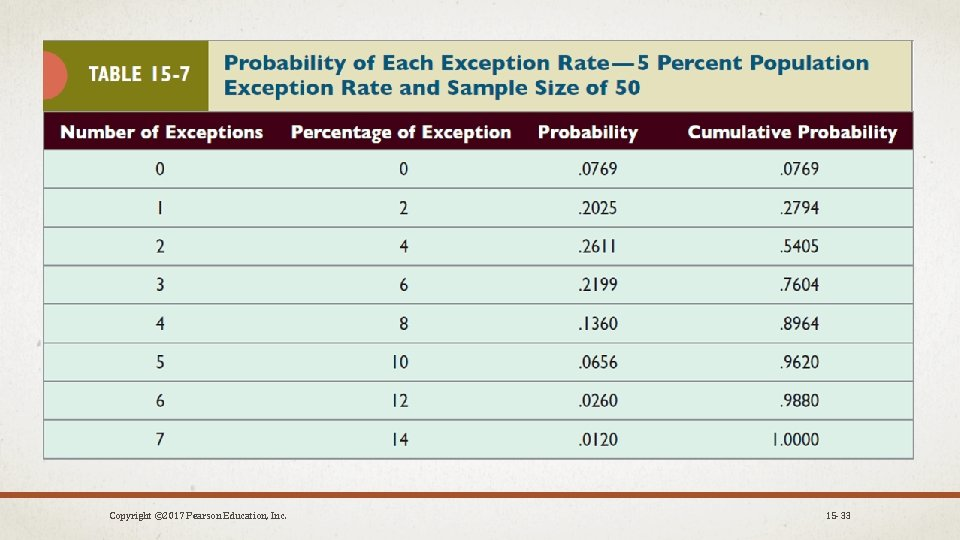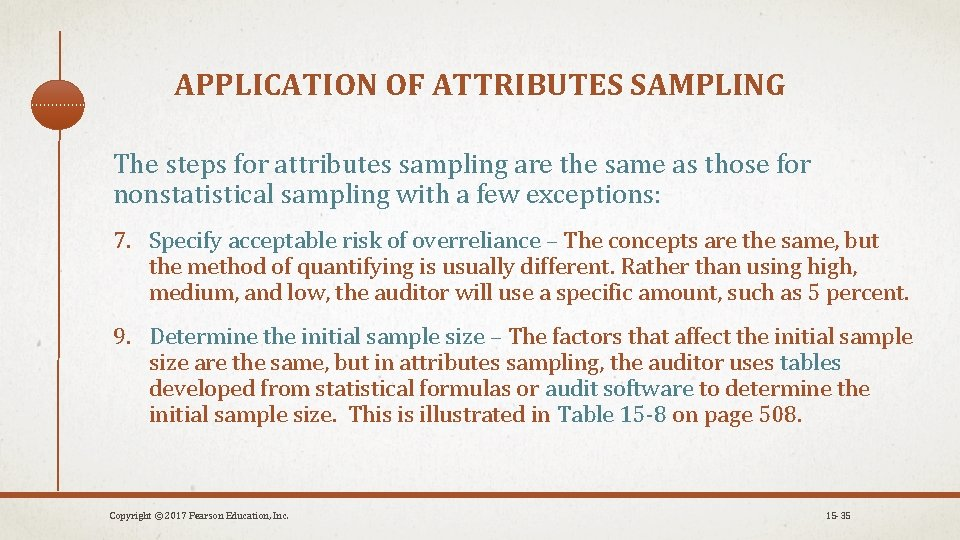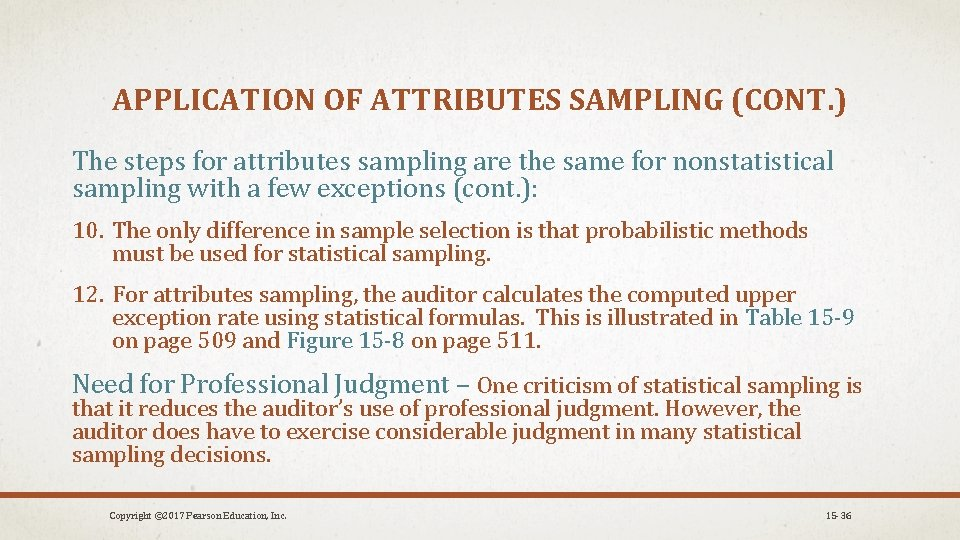# REPRESENTATIVE SAMPLING A representative sample is one in

• Slides: 36REPRESENTATIVE SAMPLING A representative sample is one in which the characteristics in the sample are approximately the same as those of the population. • Auditors strive to get a representative sample for testing. • Auditors never know if a sample is representative or not, but the auditor can increase the likelihood by using care in designing the sampling process, sample selection, and evaluation of sample results. A sample, even a representative sample, has the risk of two types of errors: a sampling error and a nonsampling error. • The risks of these two types of errors are called sampling risk and nonsampling risk. Copyright © 2017 Pearson Education, Inc. 15 -1REPRESENTATIVE SAMPLING (CONT. ) Sampling risk is the risk that an auditor reaches an incorrect conclusion because the sample is not representative of the population. Sampling risk can be controlled by the auditor by: 1. Adjusting sampling size 2. Using an appropriate method of selecting sample items from the population. Nonsampling risk is the risk that the auditor reaches an incorrect conclusion for any reason not related to sampling risk. Common reasons are: • Auditor’s failure to recognize exceptions • Ineffective audit procedures Copyright © 2017 Pearson Education, Inc. 15 -2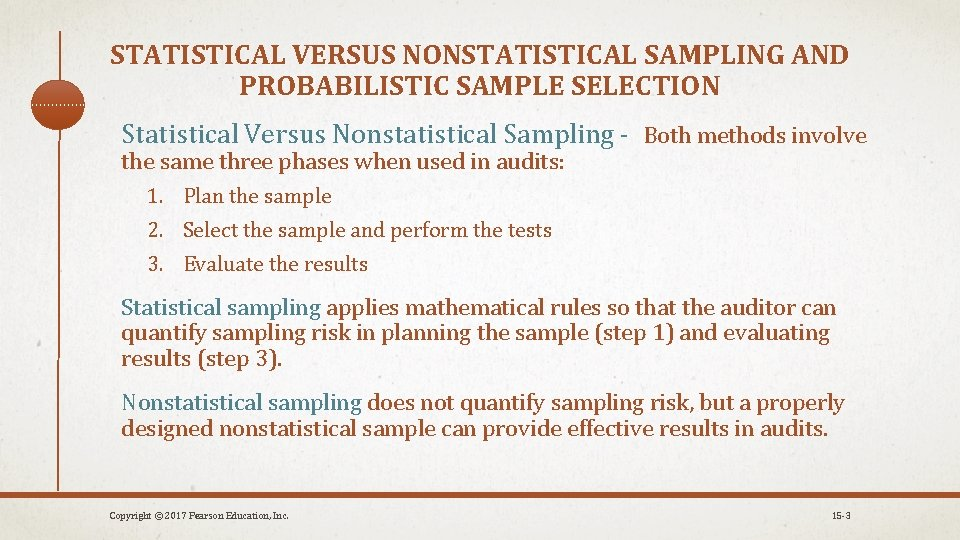STATISTICAL VERSUS NONSTATISTICAL SAMPLING AND PROBABILISTIC SAMPLE SELECTION Statistical Versus Nonstatistical Sampling - Both methods involve the same three phases when used in audits: 1. Plan the sample 2. Select the sample and perform the tests 3. Evaluate the results Statistical sampling applies mathematical rules so that the auditor can quantify sampling risk in planning the sample (step 1) and evaluating results (step 3). Nonstatistical sampling does not quantify sampling risk, but a properly designed nonstatistical sample can provide effective results in audits. Copyright © 2017 Pearson Education, Inc. 15 -3STATISTICAL VERSUS NONSTATISTICAL SAMPLING AND PROBABILISTIC SAMPLE SELECTION(CONT. ) Probabilistic Versus Nonprobabilistic Sample Selection Probabilistic sample selection – the auditor randomly selects items such that each item has a known probability of being included in the sample. • When statistical sampling is used, a probabilistic sample must be used. Nonprobabilistic sample selection – any other method of selecting a sample. Copyright © 2017 Pearson Education, Inc. 15 -4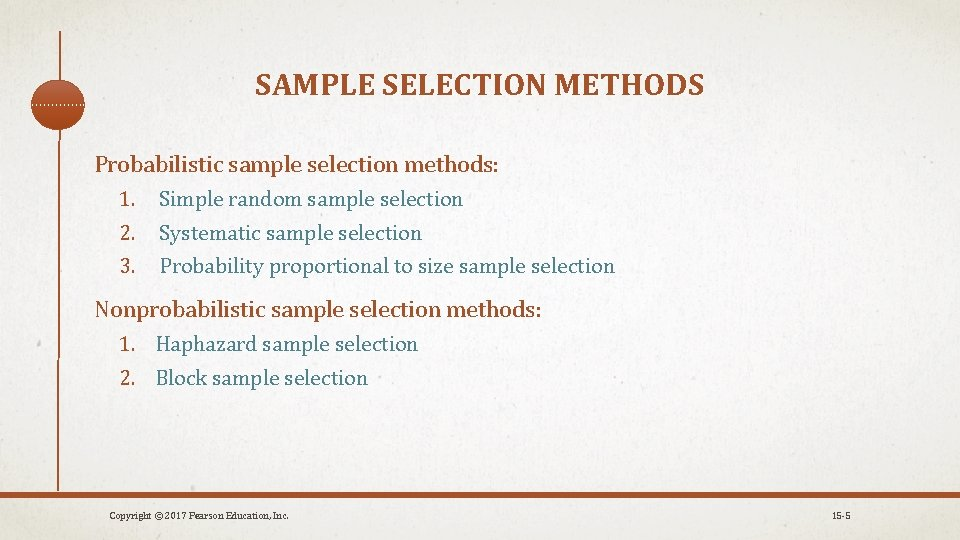SAMPLE SELECTION METHODS Probabilistic sample selection methods: 1. Simple random sample selection 2. Systematic sample selection 3. Probability proportional to size sample selection Nonprobabilistic sample selection methods: 1. Haphazard sample selection 2. Block sample selection Copyright © 2017 Pearson Education, Inc. 15 -5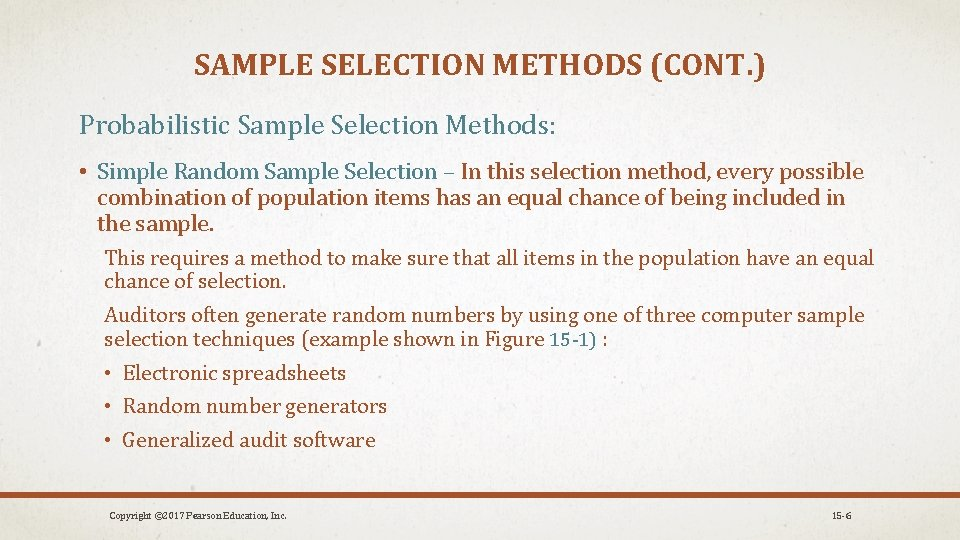SAMPLE SELECTION METHODS (CONT. ) Probabilistic Sample Selection Methods: • Simple Random Sample Selection – In this selection method, every possible combination of population items has an equal chance of being included in the sample. This requires a method to make sure that all items in the population have an equal chance of selection. Auditors often generate random numbers by using one of three computer sample selection techniques (example shown in Figure 15 -1) : • Electronic spreadsheets • Random number generators • Generalized audit software Copyright © 2017 Pearson Education, Inc. 15 -6SAMPLE SELECTION METHODS (CONT. ) Probabilistic Sample Selection Methods (cont. ): • Systematic Sample Selection • The auditor calculates an interval and then selects items for the sample based on the size of the interval. • The interval is determined by dividing the population by the desired sample size. • Probability Proportional to Size and Stratified Sample Selection • In many auditing situations, it is advantageous to select samples that emphasize population items with larger recorded amounts. Copyright © 2017 Pearson Education, Inc. 15 -8SAMPLE SELECTION METHODS (CONT. ) Nonprobabilistic Sample Selection Methods: • Haphazard Sample Selection • the selection of items without any conscious bias by the auditor • shortcoming is the difficulty of remaining completely unbiased • Block Sample Selection • the auditor selects the first item in a block and then the remainder of the block is chosen in sequence • acceptable to use block sampling if a reasonable number of blocks is used Although these methods seem less logical than probabilistic methods, there auditing situations in which a probabilistic method is not practical, such as in the situation of auditing a population that is not numbered in any way. Copyright © 2017 Pearson Education, Inc. 15 -9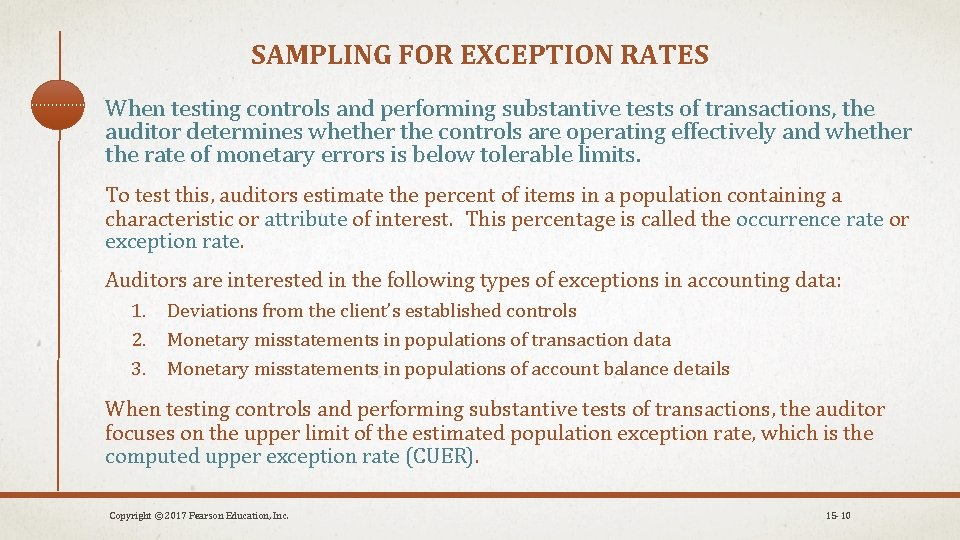SAMPLING FOR EXCEPTION RATES When testing controls and performing substantive tests of transactions, the auditor determines whether the controls are operating effectively and whether the rate of monetary errors is below tolerable limits. To test this, auditors estimate the percent of items in a population containing a characteristic or attribute of interest. This percentage is called the occurrence rate or exception rate. Auditors are interested in the following types of exceptions in accounting data: 1. Deviations from the client’s established controls 2. Monetary misstatements in populations of transaction data 3. Monetary misstatements in populations of account balance details When testing controls and performing substantive tests of transactions, the auditor focuses on the upper limit of the estimated population exception rate, which is the computed upper exception rate (CUER). Copyright © 2017 Pearson Education, Inc. 15 -10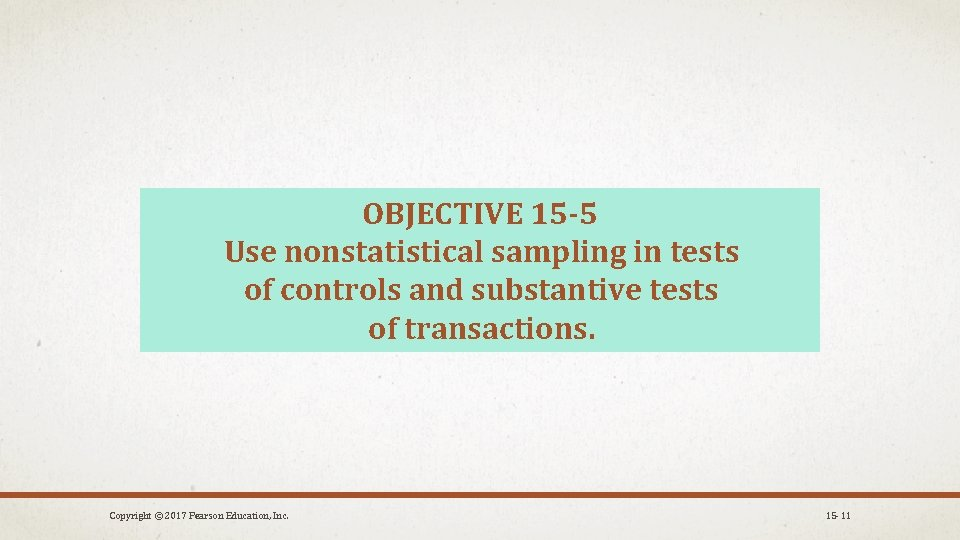OBJECTIVE 15 -5 Use nonstatistical sampling in tests of controls and substantive tests of transactions. Copyright © 2017 Pearson Education, Inc. 15 -11APPLICATION OF NONSTATISTICAL AUDIT SAMPLING The three phases of sampling are identical for both statistical and nonstatistical sampling: 1. Plan the Sample 2. Select the Sample and Perform the Audit Procedures 3. Evaluate the Results There are 14 well-defined steps that fall under the three phases. The terms used in audit sampling are detailed in Table 15 -1. Copyright © 2017 Pearson Education, Inc. 15 -12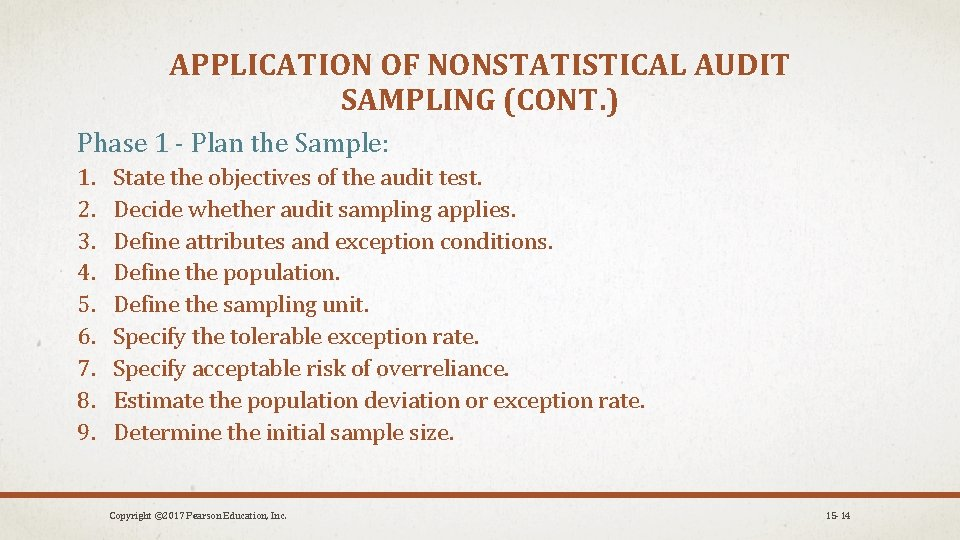APPLICATION OF NONSTATISTICAL AUDIT SAMPLING (CONT. ) Phase 1 - Plan the Sample: 1. 2. 3. 4. 5. 6. 7. 8. 9. State the objectives of the audit test. Decide whether audit sampling applies. Define attributes and exception conditions. Define the population. Define the sampling unit. Specify the tolerable exception rate. Specify acceptable risk of overreliance. Estimate the population deviation or exception rate. Determine the initial sample size. Copyright © 2017 Pearson Education, Inc. 15 -14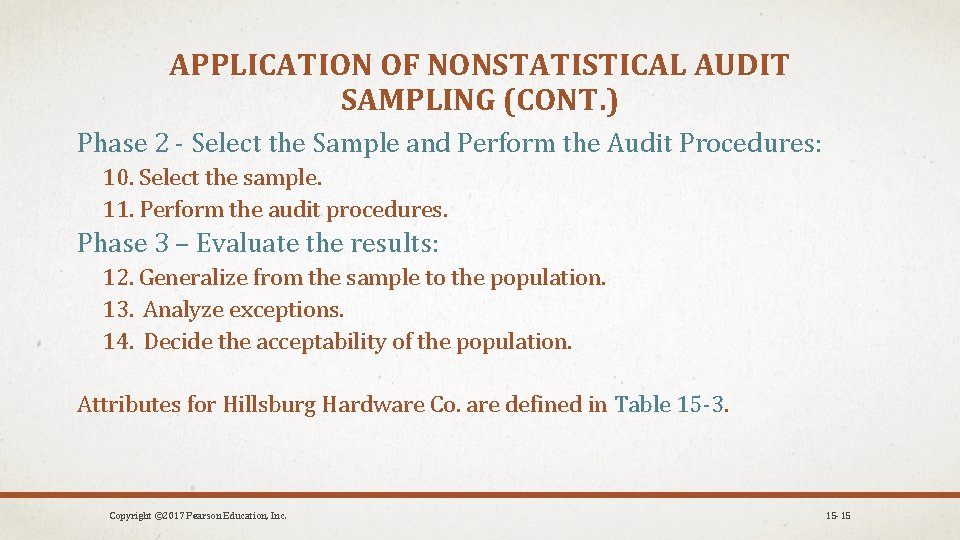APPLICATION OF NONSTATISTICAL AUDIT SAMPLING (CONT. ) Phase 2 - Select the Sample and Perform the Audit Procedures: 10. Select the sample. 11. Perform the audit procedures. Phase 3 – Evaluate the results: 12. Generalize from the sample to the population. 13. Analyze exceptions. 14. Decide the acceptability of the population. Attributes for Hillsburg Hardware Co. are defined in Table 15 -3. Copyright © 2017 Pearson Education, Inc. 15 -15APPLICATION OF NONSTATISTICAL AUDIT SAMPLING (CONT. ) Phase 1 - Plan the Sample: 1. State the Objectives of the Audit Test: The objectives of the test must be in terms of the transaction cycle being tested. The objectives are usually defined as follows: • Test the operating effectiveness of controls • Determine whether the transactions contain monetary misstatements 2. Decide Whether Audit Sampling Applies: Audit sampling applies whenever the auditor plans to reach conclusions about a population based on a sample. Audit procedures for Hillsburg Hardware Co. where audit sampling is appropriate are illustrated in Table 15 -2. Copyright © 2017 Pearson Education, Inc. 15 -16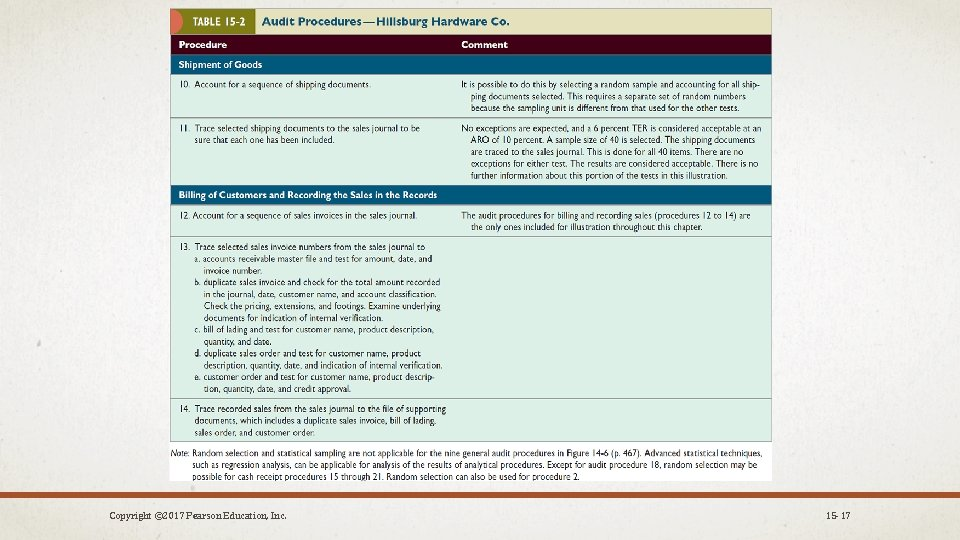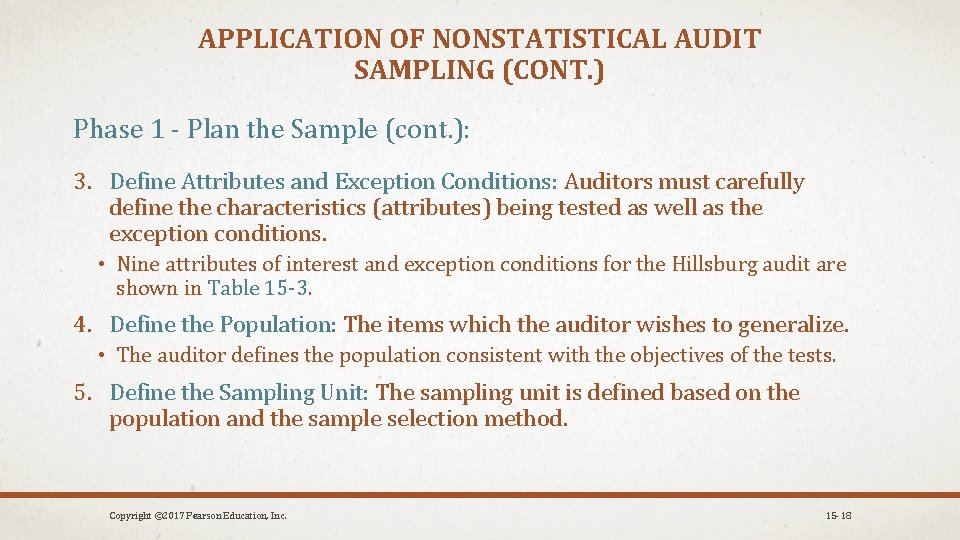APPLICATION OF NONSTATISTICAL AUDIT SAMPLING (CONT. ) Phase 1 - Plan the Sample (cont. ): 3. Define Attributes and Exception Conditions: Auditors must carefully define the characteristics (attributes) being tested as well as the exception conditions. • Nine attributes of interest and exception conditions for the Hillsburg audit are shown in Table 15 -3. 4. Define the Population: The items which the auditor wishes to generalize. • The auditor defines the population consistent with the objectives of the tests. 5. Define the Sampling Unit: The sampling unit is defined based on the population and the sample selection method. Copyright © 2017 Pearson Education, Inc. 15 -18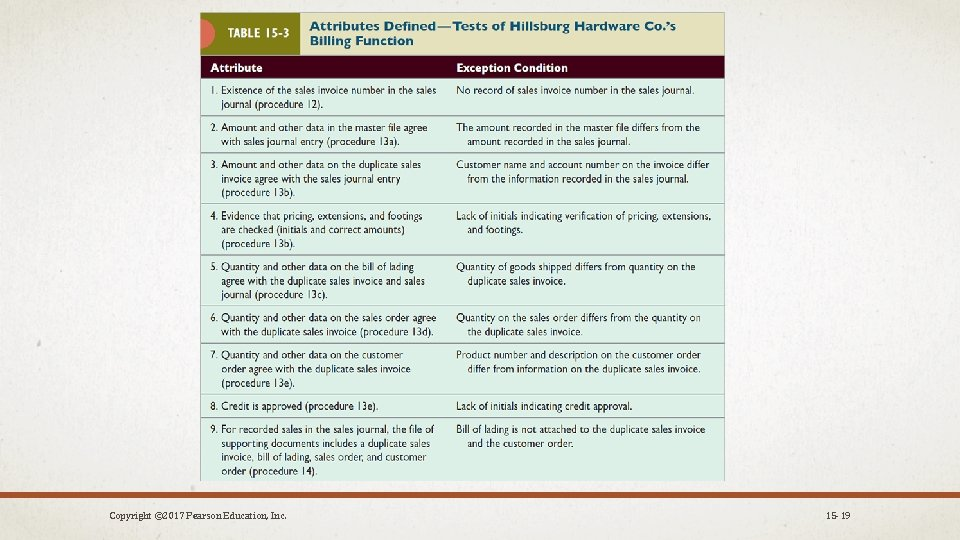APPLICATION OF NONSTATISTICAL AUDIT SAMPLING (CONT. ) Phase 1 - Plan the Sample (cont. ): 6. Specify the Tolerable Exception Rate: The tolerable exception rate (TER) for each attribute requires the auditor’s professional judgment. The TER can significantly affect the sample size. Figure 15 -2 on page 494 shows the TER on a sampling data sheet. 7. Specify Acceptable Risk of Overreliance: Sampling always has a risk of incorrect conclusions about the population. Auditors are concerned with the risk of overreliance because it impacts the effectiveness of the audit. The acceptable risk of overreliance (ARO) measures the risk the auditor is willing to take of concluding that a control is effective when the true population exception rate is greater than TER. Guidelines for establishing TER and ARO are illustrated in Tables 15 -4 and 15 -5. Copyright © 2017 Pearson Education, Inc. 15 -20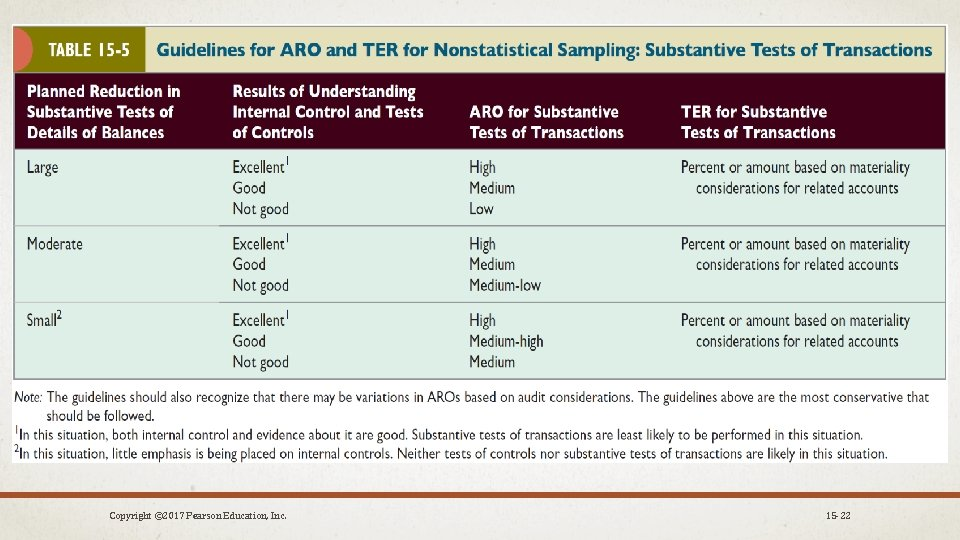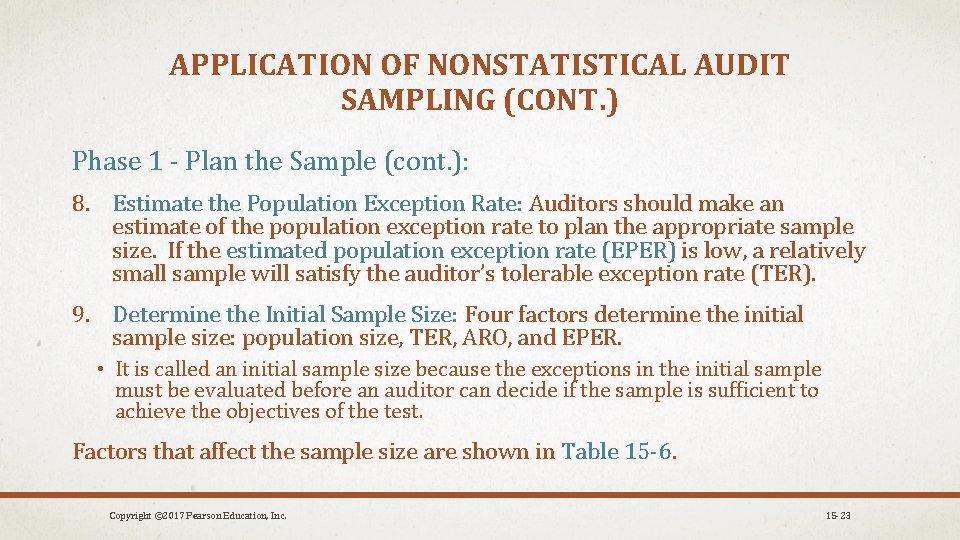APPLICATION OF NONSTATISTICAL AUDIT SAMPLING (CONT. ) Phase 1 - Plan the Sample (cont. ): 8. Estimate the Population Exception Rate: Auditors should make an estimate of the population exception rate to plan the appropriate sample size. If the estimated population exception rate (EPER) is low, a relatively small sample will satisfy the auditor’s tolerable exception rate (TER). 9. Determine the Initial Sample Size: Four factors determine the initial sample size: population size, TER, ARO, and EPER. • It is called an initial sample size because the exceptions in the initial sample must be evaluated before an auditor can decide if the sample is sufficient to achieve the objectives of the test. Factors that affect the sample size are shown in Table 15 -6. Copyright © 2017 Pearson Education, Inc. 15 -23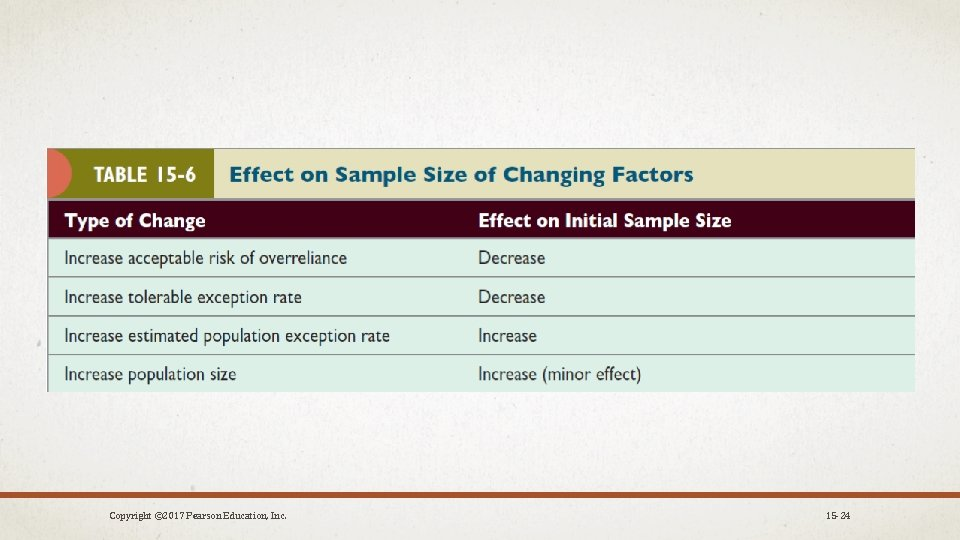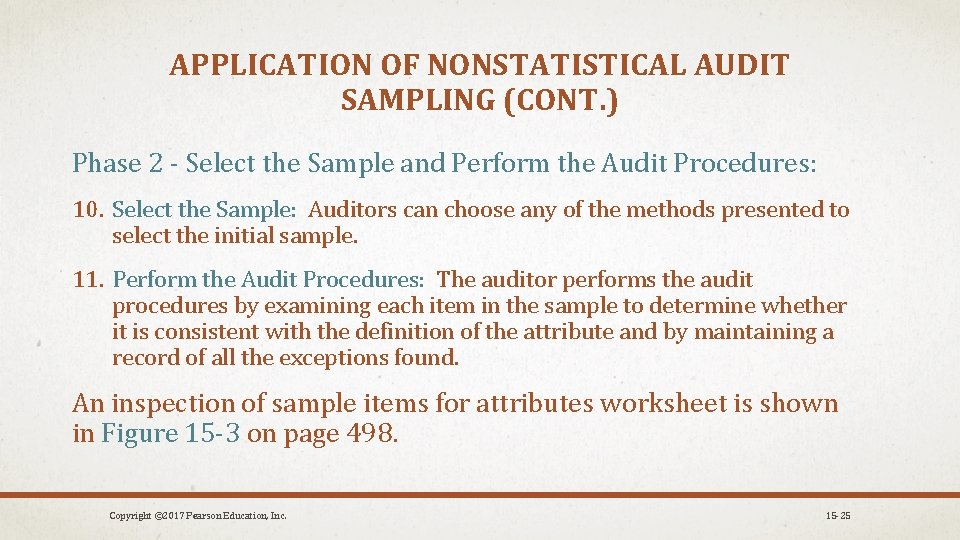APPLICATION OF NONSTATISTICAL AUDIT SAMPLING (CONT. ) Phase 2 - Select the Sample and Perform the Audit Procedures: 10. Select the Sample: Auditors can choose any of the methods presented to select the initial sample. 11. Perform the Audit Procedures: The auditor performs the audit procedures by examining each item in the sample to determine whether it is consistent with the definition of the attribute and by maintaining a record of all the exceptions found. An inspection of sample items for attributes worksheet is shown in Figure 15 -3 on page 498. Copyright © 2017 Pearson Education, Inc. 15 -25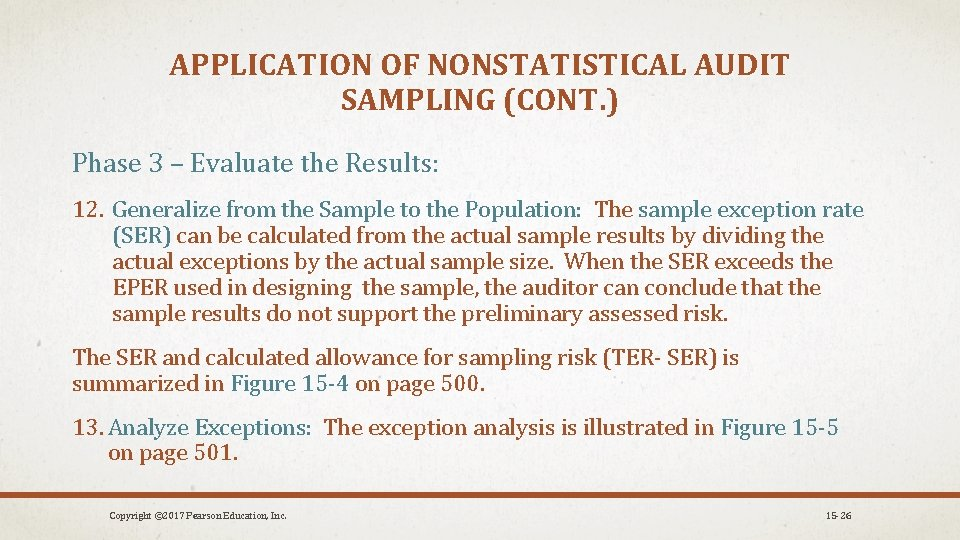APPLICATION OF NONSTATISTICAL AUDIT SAMPLING (CONT. ) Phase 3 – Evaluate the Results: 12. Generalize from the Sample to the Population: The sample exception rate (SER) can be calculated from the actual sample results by dividing the actual exceptions by the actual sample size. When the SER exceeds the EPER used in designing the sample, the auditor can conclude that the sample results do not support the preliminary assessed risk. The SER and calculated allowance for sampling risk (TER- SER) is summarized in Figure 15 -4 on page 500. 13. Analyze Exceptions: The exception analysis is illustrated in Figure 15 -5 on page 501. Copyright © 2017 Pearson Education, Inc. 15 -26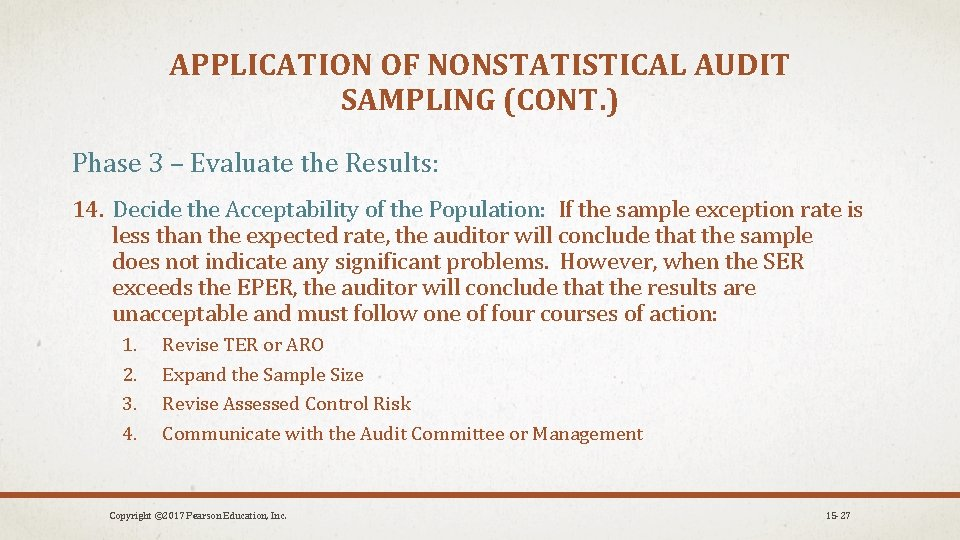APPLICATION OF NONSTATISTICAL AUDIT SAMPLING (CONT. ) Phase 3 – Evaluate the Results: 14. Decide the Acceptability of the Population: If the sample exception rate is less than the expected rate, the auditor will conclude that the sample does not indicate any significant problems. However, when the SER exceeds the EPER, the auditor will conclude that the results are unacceptable and must follow one of four courses of action: 1. 2. 3. 4. Revise TER or ARO Expand the Sample Size Revise Assessed Control Risk Communicate with the Audit Committee or Management Copyright © 2017 Pearson Education, Inc. 15 -27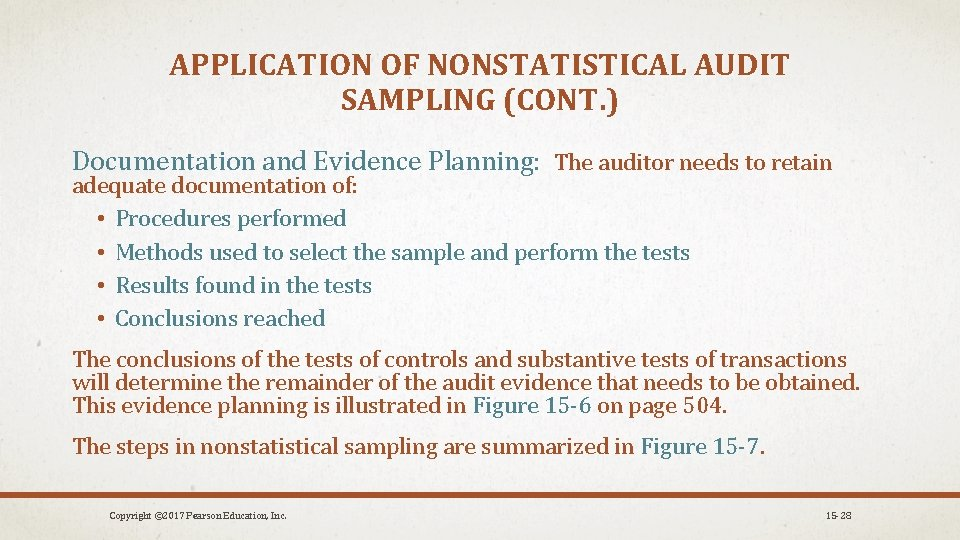APPLICATION OF NONSTATISTICAL AUDIT SAMPLING (CONT. ) Documentation and Evidence Planning: The auditor needs to retain adequate documentation of: • Procedures performed • Methods used to select the sample and perform the tests • Results found in the tests • Conclusions reached The conclusions of the tests of controls and substantive tests of transactions will determine the remainder of the audit evidence that needs to be obtained. This evidence planning is illustrated in Figure 15 -6 on page 504. The steps in nonstatistical sampling are summarized in Figure 15 -7. Copyright © 2017 Pearson Education, Inc. 15 -28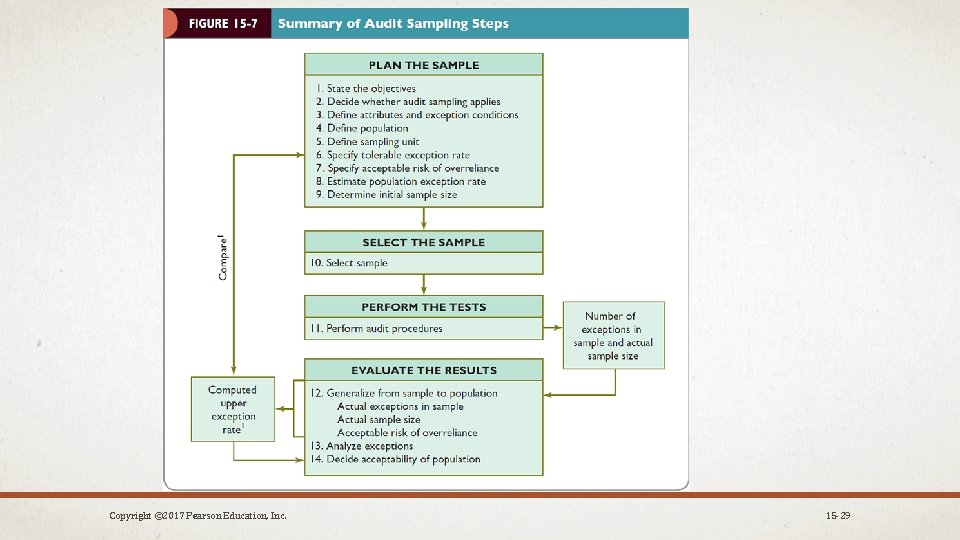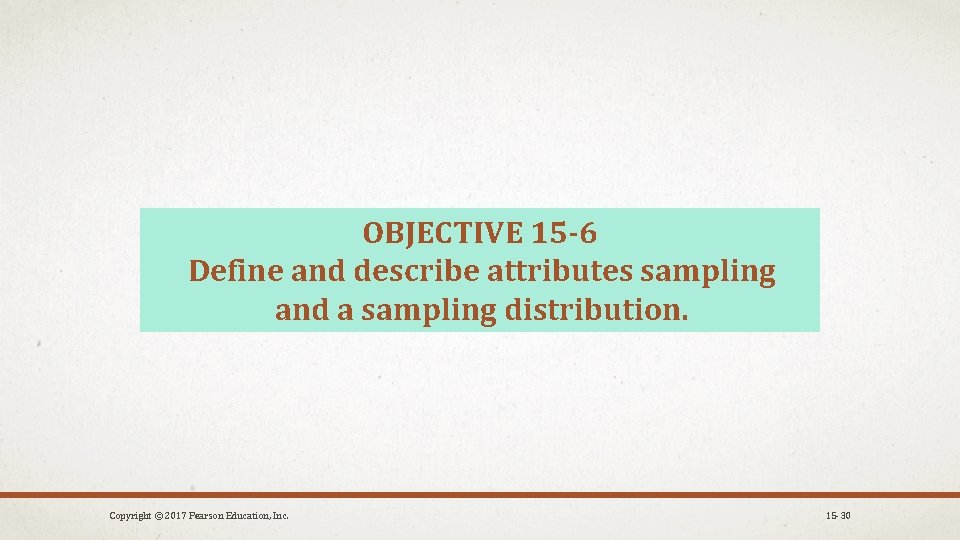OBJECTIVE 15 -6 Define and describe attributes sampling and a sampling distribution. Copyright © 2017 Pearson Education, Inc. 15 -30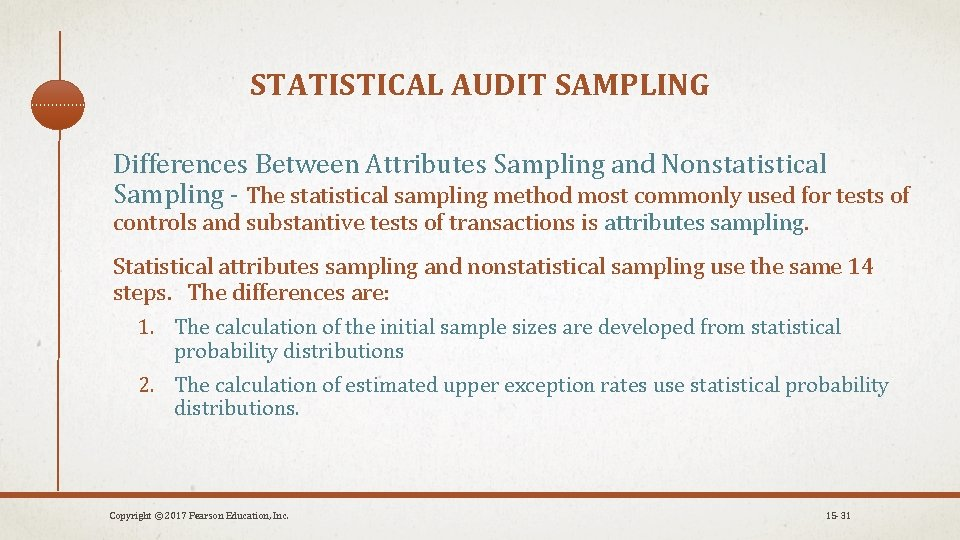STATISTICAL AUDIT SAMPLING Differences Between Attributes Sampling and Nonstatistical Sampling - The statistical sampling method most commonly used for tests of controls and substantive tests of transactions is attributes sampling. Statistical attributes sampling and nonstatistical sampling use the same 14 steps. The differences are: 1. The calculation of the initial sample sizes are developed from statistical probability distributions 2. The calculation of estimated upper exception rates use statistical probability distributions. Copyright © 2017 Pearson Education, Inc. 15 -31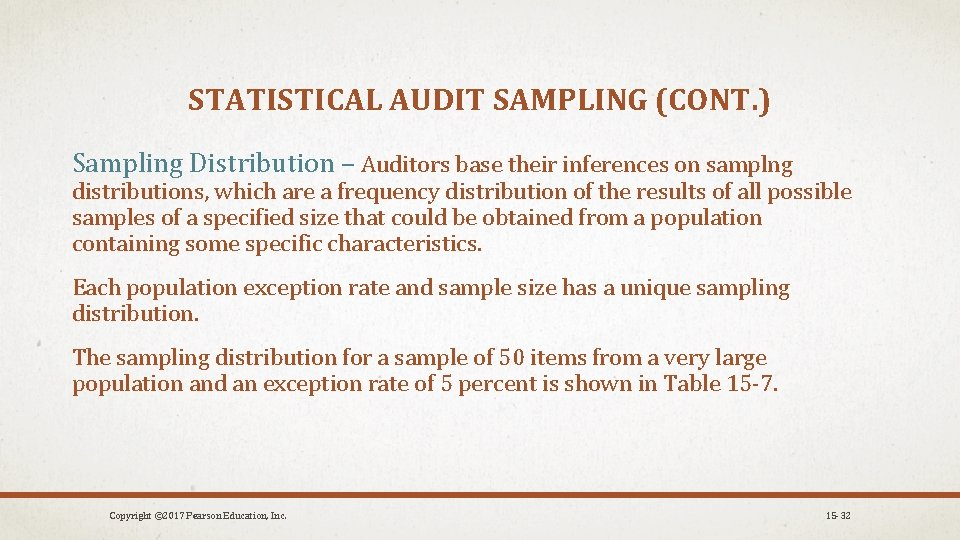STATISTICAL AUDIT SAMPLING (CONT. ) Sampling Distribution – Auditors base their inferences on samplng distributions, which are a frequency distribution of the results of all possible samples of a specified size that could be obtained from a population containing some specific characteristics. Each population exception rate and sample size has a unique sampling distribution. The sampling distribution for a sample of 50 items from a very large population and an exception rate of 5 percent is shown in Table 15 -7. Copyright © 2017 Pearson Education, Inc. 15 -32